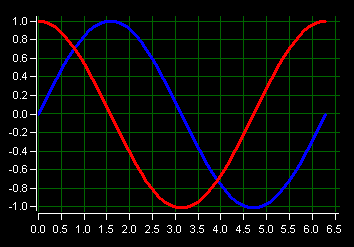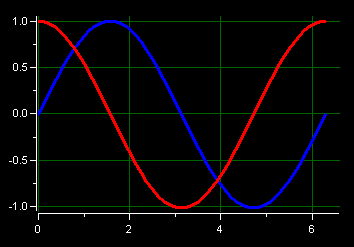# TickLabelSpacing

For X scales, TickLabelSpacing is the minimum tick label spacing based on the percentage of the maximum displayed tick label width.  The actual spacing may be greater than the minimum value depending on the major tick scale interval that is calculated.  The spacing is based on the number of characters in the major tick labels as well as the tick label font.  A value of 0 sets the spacing to be as close as possible without any of the X scale tick labels overlapping.  A value of 2 sets the spacing to be a minimum of twice the width of the widest X scale tick label.  Increasing the TickLabelSpacing value will minimize scale flicker as the auto-scale calculation determines the optimum difference between two major ticks.  Increasing TickLabelSpacing is typical for the X scale in a trending application.

For Y scales, TickLabelSpacing is the minimum vertical spacing between two major tick labels.  The actual spacing may be greater than the minimum value depending on the major tick scale interval that is calculated.  The spacing is a percentage of the tick label character height, which is based on the tick label font.  A value of 0 sets the spacing to be as close as possible without overlapping of the major tick labels.  A value of 2 sets the spacing to be a minimum of twice the height of the Y scale tick label font.  Typically in trending applications, increasing the Tick Label Spacing value minimizes scale adjustments as the auto-scale calculation determines the optimum major tick scale interval.

ExamplesYScale(1).TickLabelSpacing = 0 XScale(1).TickLabelSpacing = 0YScale(1).TickLabelSpacing = 2 XScale(1).TickLabelSpacing = 2